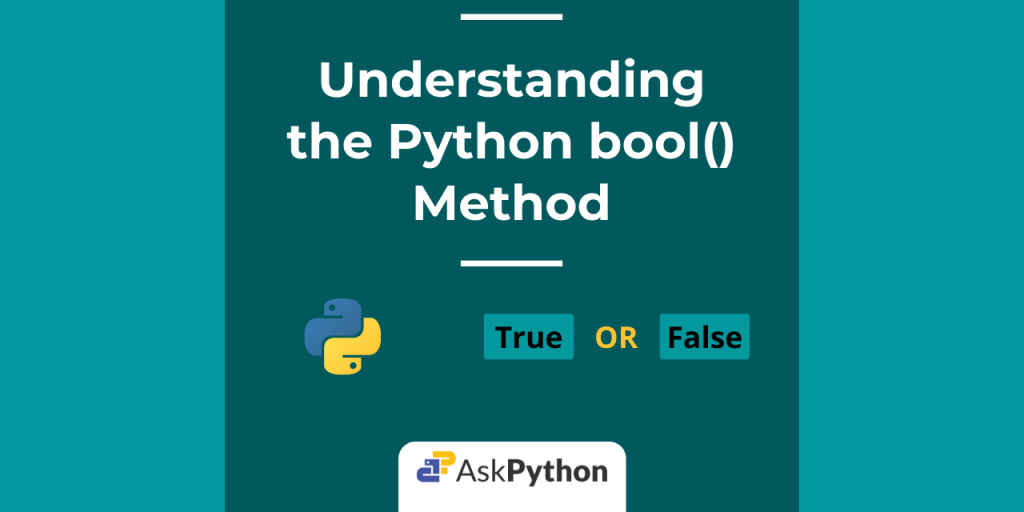# Python bool() Method: Everything You Wanted to KnowHey there! Today in this tutorial we are going to learn about the Python bool() method.

So, let us get right into the topic.

## The Python bool() Method

The bool() method is a built-in Python method that applies the standard truth testing procedure to the passed object/value and returns a boolean value. Moreover, the bool class cannot be sub-classed. Its only instances are `False` and `True`. The syntax for using the method is given below.

```bool([x])
```

Here, ` x ` is an optional parameter that can be an object, some value or any expression. The method returns `True` when True is passed and similarly returns `False` for a False.

The `bool()` method returns `False` for the below-mentioned conditions. Otherwise, it returns `True`.

• If the object has a defined __bool__() method, then the boolean result depends on what it returns. Or else if the object has __len__() defined instead of __bool__(), it’s return value is considered.
• If the value is zero of any type(0, 0.0, 0j, etc.),
• If the object is an empty collection or sequence like list, string, tuple, dictionary, etc.
• If the value is `False` or `None` constant.

Note: If for any object `x`, the` __bool__()` or `__len__()` methods are not defined, we get `True` as a result.

## Python bool() Working

Now that we have learnt the basics for the `bool()` method, let us try out some examples to get a better understanding.

### 1. With Numbers

The example below illustrates the working of the `bool()` method with numbers.

```from fractions import Fraction
from decimal import Decimal

# variable
val = 55
val1 = 0
print("bool(val):", bool(val))
print("bool(val1):", bool(val1))

# expression
print("bool(20 - 4):", bool(20 - 4))
print("bool(20 - 20):", bool(20 - 20))

# float
print("bool(0.0001):", bool(0.0001))
print("bool(0.00):", bool(0.00))

# hex
print("bool(0xF):", bool(0xF))

# Complex Numbers
print("bool(12 - 34j):", bool(12 - 34j))
print("bool(0j):", bool(0j))

# decimal floating point and fractional numbers
print("bool(Decimal(0)):", bool(Decimal(0)))
print("bool(Fraction(0, 2)):", bool(Fraction(0, 2)))
```

Output:

```bool(val): True
bool(val1): False
bool(20 - 4): True
bool(20 - 20): False
bool(0.0001): True
bool(0.00): False
bool(0xF): True
bool(12 - 34j): True
bool(0j): False
bool(Decimal(0)): False
bool(Fraction(0, 2)): False
```

### 2. With Strings

For strings, the `bool()` method returns True until an unless it’s `len()` is equal to zero(0).

```# bool() with strings

string1 = "Python"  # len > 0
print(bool(string1))

string1 = ''  # len = 0
print(bool(string1))

string1 = 'False'  # len > 0
print(bool(string1))

string1 = '0'  # len > 0
print(bool(string1))
```

Output:

```True
False
True
True
```

### 3. With built-in Objects

For sequences or collections, the method returns `False` only if the passed object is empty.

```# list
a = [1, 2, 3, 4]
print(bool(a))

# empty objects
a = []
print(bool(a))

a = ()
print(bool(a))

a = {}
print(bool(a))
```

Output:

```True
False
False
False
```

### 4. With Custom Objects

In the example below, we have defined both `__init__()` and `__bool__()` methods for our `custom` class. We construct two objects x and y with different values.

Note: Even if we had defined `__len__()` for our custom class, it won’t affect the `bool()` result as we already have `__bool__()` defined. __len_()’s return value is only considered when a class does not have it’s __bool__() defined.

```class custom():
val = 0
def __init__(self, num):
self.val = num
def __bool__(self):
return bool(self.val)

# custom objects
x = custom(0)
y = custom(52)

print(bool(x))
print(bool(y))
```

Output:

```False
True
```

Here, the `bool()` results for both custom objects `x` and `y` are indirectly dependent on the passed arguments(0 for x, 52 for y). Hence, we get `False` for x(bool(0) = False) and `True` for y(bool(52) = True).

## Wrapping Up

That’s it for today. Hope you had a clear understanding of the topic – bool() method in Python. We recommend going through the links mentioned in the references section for more info on the topic.

For any further questions, feel free to use the comments below.